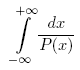ENG  RUS Timus Online JudgeOnline Judge
Problems
Authors
Online contests
Site news
Webboard
Problem set
Submit solution
Judge status
Guide
Register
Authors ranklist
Current contest
Scheduled contests
Past contests
Rules

Contest is over

D. Definite Integral

Time limit: 1.0 second
Memory limit: 64 MB
Your task is to evaluate the following definite integral:where P(x) = a4 · x4 + a3 · x3 + a2 · x2 + a1 · x + a0. P(x) has no real roots and GCD(P(x), P'(x)) = const.

Input

The input contains five integers: a0, a1, a2, a3 and a4 separated by whitespace. Each of these numbers does not exceed 106 by absolute value, a4 ≠ 0.

Output

Output the value of the integral. Assume that the exact value is A and your answer is B. Your answer will be considered correct if and only if at least one of the following statements is true:
• A − 10−9BA + 10−9
• A · (1 − 10−9) ≤ BA · (1 + 10−9)

Sample

inputoutput
16 0 0 0 1
0.2776801836
Problem Source: SPbSU ITMO contest. Petrozavodsk training camp. Winter 2008.
To submit the solution for this problem go to the Problem set: 1621. Definite Integral